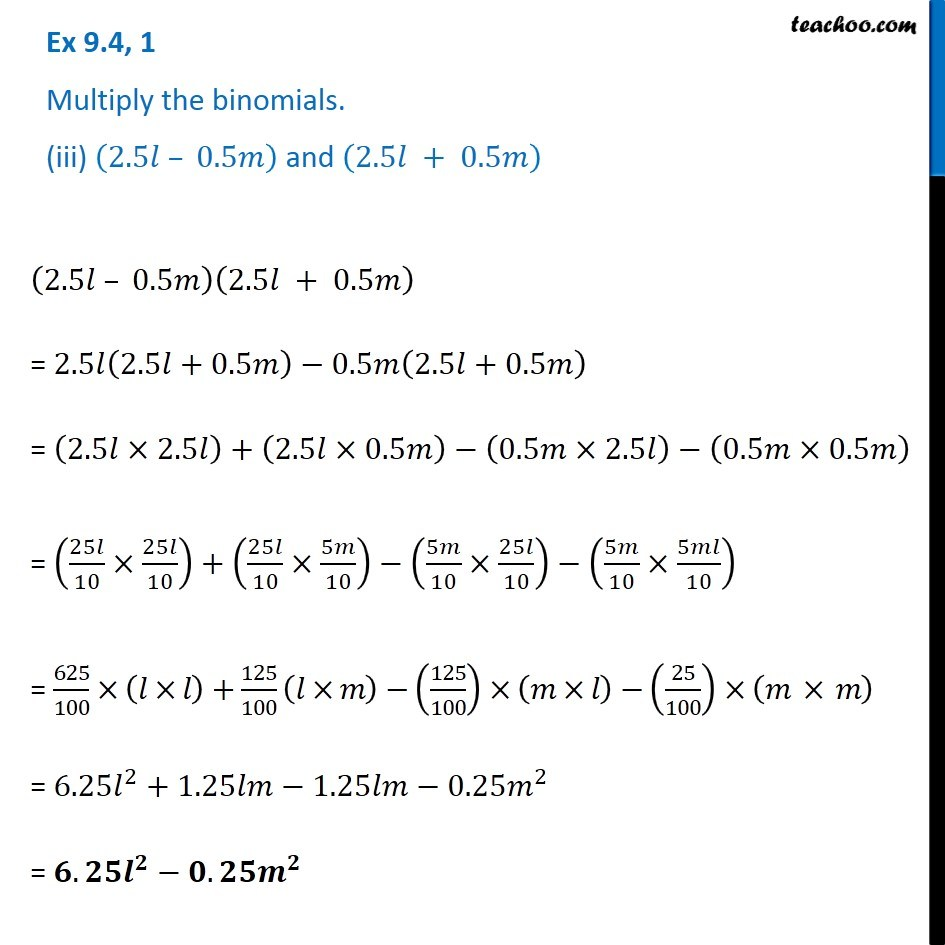1. Chapter 9 Class 8 Algebraic Expressions and Identities
2. Serial order wise
3. Ex 9.4

Transcript

Ex 9.4, 1 Multiply the binomials. (iii) (2.5𝑙 – 0.5𝑚) and (2.5𝑙 + 0.5𝑚) (2.5𝑙 – 0.5𝑚)(2.5𝑙 + 0.5𝑚) = 2.5𝑙(2.5𝑙+0.5𝑚)−0.5𝑚(2.5𝑙+0.5𝑚) = (2.5𝑙×2.5𝑙)+(2.5𝑙×0.5𝑚)−(0.5𝑚×2.5𝑙)−(0.5𝑚×0.5𝑚) = (25𝑙/10×25𝑙/10)+(25𝑙/10×5𝑚/10)−(5𝑚/10×25𝑙/10)−(5𝑚/10×5𝑚𝑙/10) = 625/100×(𝑙×𝑙)+125/100 (𝑙×𝑚)−(125/100)×(𝑚×𝑙)−(25/100)×(𝑚 × 𝑚) = 6.25𝑙^2+1.25𝑙𝑚−1.25𝑙𝑚−0.25𝑚^2 = 𝟔.𝟐𝟓𝒍^𝟐−𝟎.𝟐𝟓𝒎^𝟐

Ex 9.4